# Why seeing is NOT believing? — the bayesian perspective!

Imagine you are on a game show. There are three closed doors in front of you. One door has a car behind it, and the other two doors have a goat. The objective is to try and choose the door that has the car (of course!). You pick a door. Before revealing what’s behind it, the game show host opens one of the two closed doors and shows you a goat. Now, he asks you a question.

“Switch or Stay”?

You have the option to stay with your original choice or switch to the other closed door. What would you do?

Well, in my mind, I am thinking, I have already picked a door and there’s another closed door. The car has to be in any one of these two doors. Hence, the probability of the car being in any one of these two doors, irrespective of whether I switch or stay is 50%. Right?

Wrong!

Most of you might know this, as the Monty Hall problem(named after the game show host). Play/simulate the “Monty Hall game” yourself and find out what works. https://www.mathwarehouse.com/monty-hall-simulation-online/

Did you notice that switching from the original choice, actually increase your chances of winning? Why is that? Hold that thought!

Let us understand the “what”, before jumping into the “hows & whys”. What happens after Monty opens one of the closed doors? We update our beliefs about the other two doors, basis the evidence presented. An everyday life example would be, changing our prior assumptions about something/someone, after we find out evidence either proving/disproving our belief. That’s the intuition behind Bayes Theorem.

Here’s the formula for the Bayes theorem:-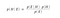Bayes Theorem

p(H|E) — posterior probability of the hypothesis H, given the evidence E . This can also be thought of as the ‘updated belief’.

p(E|H) — likelihood to see the evidence E, given the hypothesis H is true.

p(H) — prior probability of the hypothesis H or the original belief, before any evidence was seen.

p(E) — probability of the evidence E, irrespective of the hypothesis being true.

Let’s look at some examples.

Probability of an event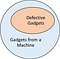Probability of an event

Eg.1 Some number of gadgets are produced from a machine, of which a few are defective.

p(Defective) = number of defective gadgets / total number of gadgets produced

That was straightforward!

Conditional Probability of an event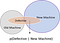Conditional Probability of an event

Eg.2 In the above example, let us assume that the gadgets are being produced from two different machines, old and new.

p(Defective | New Machine) = number of defective gadgets produced by the new machine / total number of gadgets produced by the new machine

That was quite intuitive too. With two machines, one being old and the another new, naturally one would expect the new machine to produce lesser defective gadgets. At the end of the production line, if a random defective gadget is found, what is the probability that it was from the new machine? This can be calculated using the Bayes theorem.

Eg.3 We know from the previous experiments, that the defect rate is 20% and 8% in the old and new machines respectively. Also, let us say that the new machine produces three times more gadgets than the old machine. To find the probability of a random gadget being produced by the new machine, given it is defective, we apply the Bayes theorem.

To calculate the probability of a defective gadget, we will have to consider the defective gadgets from both the old and new machines. Hence, we re-write the formula as below.

The probability that a random defective gadget being produced from the new machine is 55%. What does this indicate? With the given defect rate, the chances are, a little over half of the total defective gadgets are from the new machine, disproving our original assumption that the new machine might produce lesser defective gadgets.

If that was, may be, just a little confusing, contingency table is here for your rescue.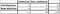Contingency Table — Bayes Theorem

Let us assume that there are a total of 100 gadgets being produced by both the old and the new machines together. Substituting the values from the problem statement, we will arrive at the contingency table (on the left).

• What’s the probability that an incoming mail is spam?:- If we know p(spam-trigger word), p(spam) and the p(spam-trigger word | spam), we can find the probability of a new mail being spam, given the occurrence of a spam-trigger word, that is, p(spam | spam-trigger word).
• Does a positive test result always indicate cancer? :- Given the p(cancer), p(positive) and the p(positive | cancer), the probability that someone has cancer, given the test result is positive, or p(cancer | positive), can be found.
• Will it rain, if its cloudy? :- In a given season/month, knowing the p(cloudiness), p(rain) and the p(cloudiness | rain), we can calculate the probability of rainfall, given it’s cloudy or p(rain | cloudiness).

You get the idea, right?

Alright! Now, we know what’s Bayes theorem. How does that apply to our original ‘Monty Hall problem’? Let’s go step by step.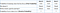Prior Probabilities — Bayes Theorem

Initially, all the doors have the same probability of 0.33. After we choose door A, Monty has a choice to make.

1. If the car was behind door A, Monty has an equal probability of choosing doors B or C, that is 0.5.
2. If the car was behind B, he could choose only C, since we’ve already chosen A. Same holds good if the car was behind C. Hence, the probability of choosing either B or C becomes 1.

In this example, we assume Monty chooses to reveal door B. We calculate the conditional probabilities of Monty opening door B, given each door has the car. The conditional probabilities are added to arrive at the probability of Monty choosing door B or the likelihood.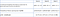Calculating Likelihood — Bayes Theorem

With the new evidence or the likelihood that Monty chooses a particular door, changes the perspective about the other two doors. The posterior probabilities of doors A & C are calculated as below.

We originally chose door A. After door B was revealed, our initial assumption was that doors A&C carry the same probability of 0.5. However, after applying Bayes Theorem, with the new information about the probability of the host choosing a particular door, we calculated the posterior probability of door A as 0.33 and door C as 0.66. Thus proving, Switching is better!

In this scenario, Monty knows behind which door is the car hidden. What happens, if Monty is unaware of that information? Will that change the posterior probability? Any thoughts?

## Analytics Vidhya

Analytics Vidhya is a community of Analytics and Data…

### By Analytics Vidhya

Latest news from Analytics Vidhya on our Hackathons and some of our best articles! Take a look.

By signing up, you will create a Medium account if you don’t already have one. Review our Privacy Policy for more information about our privacy practices.

Check your inbox
Medium sent you an email at to complete your subscription.

## Analytics Vidhya

Analytics Vidhya is a community of Analytics and Data Science professionals. We are building the next-gen data science ecosystem https://www.analyticsvidhya.com

Written by

## Dhivya Karthic

Data Science Enthusiast | https://www.linkedin.com/in/dhivyakarthic/## Analytics Vidhya

Analytics Vidhya is a community of Analytics and Data Science professionals. We are building the next-gen data science ecosystem https://www.analyticsvidhya.com

Medium is an open platform where 170 million readers come to find insightful and dynamic thinking. Here, expert and undiscovered voices alike dive into the heart of any topic and bring new ideas to the surface. Learn more

Follow the writers, publications, and topics that matter to you, and you’ll see them on your homepage and in your inbox. Explore

If you have a story to tell, knowledge to share, or a perspective to offer — welcome home. It’s easy and free to post your thinking on any topic. Write on Medium